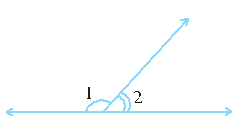# Ex.5.1 Q6 Lines and Angles - NCERT Maths Class 7

## Question

In the given figure, $$\angle 1$$ and $$\angle 2$$ are supplementary angles. If  $$\angle 1$$ is decreased, what changes should take place in $$\angle 2$$ so that both the angles remain supplementary?Video Solution
Lines & Angles
Ex 5.1 | Question 6

## Text Solution

What is known?

Two angles which are equal to its supplementary.

What is the unknown?

Change in one of the angles if other is decreased provided both angles still remain supplementary.

Reasoning:

Let’s solve this problem visually. There are two operations done in sequence. First, if one angle is decreasing then another angle will increase but their sum will remain same i.e. $$180^\circ.$$

According to this model, the resultant sum of angle $$\angle 1$$ and $$\angle 2$$ will remain $$180^\circ.$$

Steps:

If $$\angle 1$$ is decreased by some degrees, then $$\angle 2$$ will also be increased with the same degree, so both the angles will remain supplementary.

Learn from the best math teachers and top your exams

• Live one on one classroom and doubt clearing
• Practice worksheets in and after class for conceptual clarity
• Personalized curriculum to keep up with school